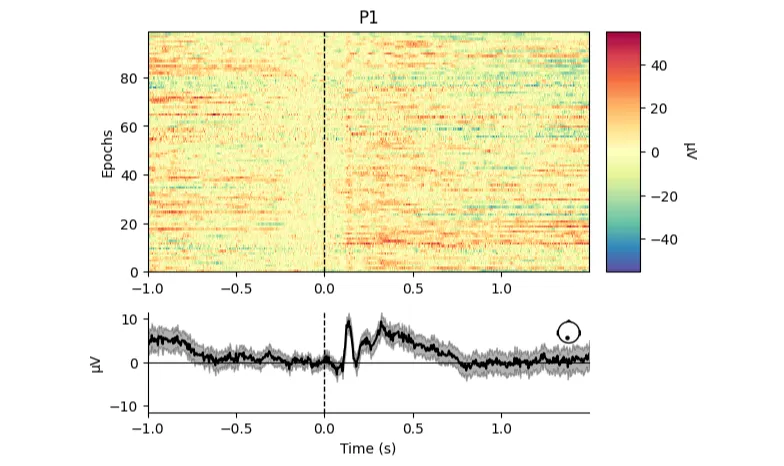## Signal Processing

Signal processing basics and EEG.## Preamble

``````import mne
``````

## Dataset

``````epochs = mne.io.read_epochs_eeglab('sampleEEGdata.mat', verbose=False)
``````

## Plotting

``````fig = epochs.plot_image(picks=['P1'],cmap='Spectral_r');
``````
```Not setting metadata
99 matching events found
No baseline correction applied
0 projection items activated
`````````fig
``````
``````epochs.plot_image(picks=['T8','T7'],cmap='Spectral_r');
``````
```Not setting metadata
99 matching events found
No baseline correction applied
0 projection items activated